## Comparison of means: the t test

A t test is used to compare the means of two data sets, and it relies on calculation of a test statistic called t. This statistic is derived from the two data sets and it is defined as the difference between the means of the two data sets, x̅1 and x̅2 (or the difference between a mean x̅ and a reference value, xref) divided by the pooled standard error of the mean(s).

The standard error is a measure of the unreliability of a statistic. The standard error of a mean is:$SE_x = \sqrt{ \frac{s^2}{n} }$

The unreliability of an estimate of the population mean increases with the variance of the sample you are using to estimate it (hence the variance, s2, in the numerator) but decreases with sample size (hence n in the denominator).

The t statistic is calculated as follows:$t = \frac{ | \bar{x}_1 - \bar{x}_2 | }{ \sqrt{ \frac{ (n_1 - 1)s_1^2 + (n_2 - 1)s_2^2 }{ n_1 + n_2 - 2 } \cdot ( \frac{1}{n_1} + \frac{1}{n_2} ) } }$

Where x̅, s and n are the mean, standard deviation and sample size of the two data sets.

A t statistic can also be used to calculated from the mean of a single data set and a reference value, xref:$t = \frac{ | \bar{x} - x_{ref} | }{ \sqrt{ \frac{s^2}{n} } }$

The reference distribution for the t test is Student’s t. Like Fisher’s F, this reference distribution has two parameters: the number of degrees of freedom remaining in the first data set, and the number of degrees of freedom remaining in the second data set. As you are comparing one mean from each data set, then the remaining degrees of freedom for each data set is n−1, and the total number of degrees of freedom is n1+n2−2.

A t test compares the t statistic from your empirical data with the Student’s t value you’d expect under the null hypothesis, given the degrees of freedom in the two data sets. The p value of the test is the probability of obtaining a test t statistic at least as extreme as the one that was actually observed, assuming that the null hypothesis (“the means are equal”) is true. i.e. The p value is the probability of observing your data (or something more extreme), if the means really are equal.

The comparison is only valid if the sample data sets are:

• Representative of the larger population.
• Normally distributed. If the normality assumption is badly violated, a non-parametric alternative such as Wilcoxon rank sum (“Mann-Whitney U“) test, using wilcox.test(), might be an appropriate alternative.
• Independent of one another. A modification of the t test can be made for certain kinds of dependent data (e.g. where the samples in each group fall into natural pairs).
• Homoscedastic; that is they have the same variance. You can test for this assumption using the F test. If the two samples have difference variances, they are heteroscedastic. The Welch modification of Student’s t test can be used if this assumption is broken.

Do not use a Student’s t test if these assumptions are broken.

In R, a t-test is performed using t.test(). Here we generate thirty items of random normal data using rnorm() and compare two such data sets. We add 10 to all the data in dataset2 to make the means differ. The output of your code will differ as the data is random (if you’re very un/lucky, the difference may not even be significant!):

dataset1 <- rnorm(30)
dataset2 <- 10 + rnorm(30)
t.test( dataset1, dataset2 )
        Welch Two Sample t-test
data: dataset1 and dataset2
t = -46.0075, df = 56.604, p-value < 2.2e-16
alternative hypothesis: true difference in means is not equal to 0
95 percent confidence interval:
-10.444369  -9.572988
sample estimates:
mean of x   mean of y
0.01425418   10.02293268

Here the p value is less than 0.05, so we can say the difference in means is significant.

In R, the default t.test() actually assumes the variances are unequal (heteroscedastic), as this is very common, and it automatically uses Welch’s modification for unequal variances, which is actually rather less gnarly to look at in equation form:$t = \frac{ | \bar{x}_1 - \bar{x}_2 | }{ \sqrt{ \frac{s_1^2}{n_1} + \frac{s_2^2}{n_2} } }$

The degrees of freedom for Welch’s modification are no longer an integer, but instead:$df = \frac{ ( s_1^2/n_1 + s_2^2/n_2 )^2 }{ \frac{(s_1^2 / n_1)^2}{ n_1 - 1} + \frac{ (s_2^2 / n_2)^2 }{ n_2 - 1} }$

You can force R to use Student’s original t test for homoscedastic data by setting the option var.equal=TRUE.

# The code below should only be used if an F test indicates the variances of
# the two data sets are equal

t.test( dataset1, dataset2, var.equal=TRUE )

# The code below has the same effect as calling
t.test( dataset1, dateset2 )

# i.e. it uses Welch's modification to account for the difference in variances
t.test( dataset1, dataset2, var.equal=FALSE)

In general, you should use an F test to see whether the variances of your two data sets are equal: if so, use Student’s t (var.equal=TRUE); if not, use Welch’s modification of t, (var.equal=FALSE).

As with plot(), it is usually more convenient to use the ‘modelled-by’ tilde ~ operator to perform t tests within named data frames, rather than supplying the test with two vectors of data.

Here we t test the dog-whelk data in dog_whelks.csv that you already made in the exercises of an earlier post. As we have already established that the variances are not significantly different, we should use Student’s t rather than the Welch modification:

dog.whelks<-read.csv( "H:/R/dog_whelks.csv" )
t.test( Height ~ Exposure, data = dog.whelks,
var.equal=TRUE )
        Two Sample t-test
data:  Height by Exposure
t = -8.6399, df = 54, p-value = 9.274e-12
alternative hypothesis: true difference in means is not equal to 0
95 percent confidence interval:
-9.989079   -6.226306
sample estimates:
mean in group Exposed   mean in group Sheltered
20.19231                28.30000

Do a t test on the data in the dog.whelks data frame, using Height as the dependent variable, and grouping the data into two sets according to the Exposure factor

You should never put raw R output into written prose. If you wish to include the results of the F and t tests on the dog-whelk data above in a report, they should be written long-hand. In typical scientific literature, this might be something like…

Dog-whelk shell-height on exposed beaches was not significantly more variable than on sheltered beaches (F=1.77, p=0.13), based on a sample size of 25. However the shells on sheltered beaches were found to be significantly taller, by 10 mm (t=6.27, p ≪0.001).

…although this style of report mixes up Fisher and Neyman-Pearson approaches to hypothesis testing: by reporting a p value, you’re probably trying to persuade people of the importance of the ‘highly significant’ t test result (Fisher); however you’re also reporting your acceptance of the alternative hypothesis (Neyman-Pearson) under which the p value is irrelevant (except insofar as it is smaller than 0.05), but the power (which you’ve not stated, let alone used to determine an appropriate sample size!) is critical to the interpretation of the results. Ho hum. To do Neyman-Pearson properly, you should do a power calculation, for which you will find the function power.t.test useful.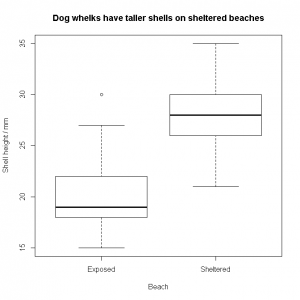You can select the heights of the sheltered-beach shells by using tapply(). This applies a function to a vector of data, grouped by another vector of the same length containing the levels of a factor.

N.B. The “t” in tapply() has nothing to do with the “t” in t– test: there are several other apply() functions with similarly cryptic names.

Remembering that the dog.whelks data frame looks like this:

   Height  Exposure
1      25  Sheltered
2      30  Sheltered
3      29  Sheltered
…
54     20   Exposed
55     24   Exposed
56     30   Exposed

We can apply the mean() function to the Height column (dog.whelks$Height) grouped by the Exposure factor (dog.whelks$Exposure) using this syntax:

# tapply( vector.to.group, factors.to.group.by, function.to.call )
means<-tapply( dog.whelks$Height, dog.whelks$Exposure, mean )
means["Sheltered"]-means["Exposed"]
Sheltered
8.107692

Shells from sheltered beaches are 8 mm taller. There are several simpler ways of working this out, e.g. using the linear model function lm(), which we will cover in later posts.

lm( Height ~ Exposure, data = dog.whelks )

To check the normality assumption for both F and t tests holds, you can use a qqnorm() plot and/or a hist() plot.

The normal quantile-quantile (QQ) plot plots the real data against theoretical normally-distributed data of the same mean and standard error. If the real data are normally distributed, then the QQ plot should be a straight line. Curved or S-shaped QQ plots are indicative of non-normal data. Note the use of the [ condition ] indexing brackets to extract out from the vector only those items for which the Exposure factor is “Sheltered“: the overall data set will certainly not be normal, as the t-test shows the two exposures come from two different (normal) distributions:

qqnorm( dog.whelks$Height[ dog.whelks$Exposure=="Sheltered" ] )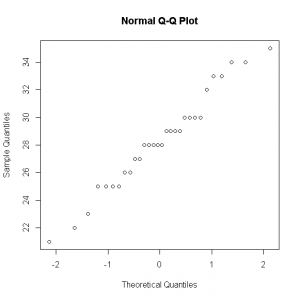A histogram of the data should look like a normal bell-curve, without obvious tails (skew) or peakiness/flatness (kurtosis); however, for small data sets, the histogram is unlikely to be smooth and ‘perfect’ looking, as you can see below. For this reason, the normal QQ plot is generally the preferred way of seeing whether data are normal enough to be analysed using F and t tests.

hist( dog.whelks$Height[dog.whelks$Exposure=="Sheltered"] )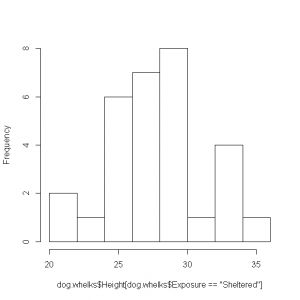You might like to plot a QQ plot and a histogram of the “Exposed” portion of the data set, and perhaps look at a histogram of the entire data set, to confirm that it is indeed composed of two overlapping (approximately) normal curves.

Some things to bear in mind when writing summaries of statistics in prose:

• Do not include raw R output.
• Include the name of the test you performed, the test statistic and its p value.
• R will usually give you the p value itself, or – as here – an indication that it is lower than the minimum value it can calculate. There is no need to additionally look up your t statistic (6.27) in tables to confirm that this is indeed associated with a p value below the critical value of your choice. This makes about as much sense as performing the calculation 34378 times 83623 on a calculator, ignoring the result, and then using a slide-rule to estimate the product instead.
• Don’t simply say a difference in means investigated by a t test is “significant”. Say which level of the factor is associated with the larger or smaller mean: it’s not just that the dog-whelk heights were different, the dog-whelks on sheltered beaches were – on average – taller. This is acceptable, as a one-tailed t test (which would test for ‘taller’ or ‘shorter’) will always have a p value less than 0.05 if the corresponding two-tailed t test  has a p value less than 0.05.
• More generally, make sure the reader knows why you’ve done the statistics: it’s too easy to forget that the whole point is to see whether some dependent variable of interest (dog-whelk shell height) can be modelled/explained using an independent variable of interest (type of beach the shell is found on).
• Give an indication of the sample size.

# Exercises

Use F and t tests to investigate the following data sets. For each data set, write a short piece of prose describing the results of the statistical analysis. Plot suitable graphs for each set to accompany the text.

1. The data in pollen_tubes.csv show the growth rate of pollen tubes from Impatiens pollen, grown in an agar-solidified basal medium containing sucrose, vs. the same medium with borate and calcium supplementation.
2. The data in pipette_check.csv show the mass (g) of water measured when two brands of 1 mL adjustable pipettes are set to their maximum volume and used to pipette pure water. Which brand of pipette is better?
3. You intend collecting some data on the growth rate of bacteria in a liquid broth culture. Due to space limitations, you can only use 8 flasks. You intend on running 4 flasks as controls, and spiking another 4 with silver nitrate to see what effect it has on the exponential growth rate. Some preliminary experiments indicate that the control growth rate is typically 0.02 min−1 with a standard deviation of 0.005 min−1. Assuming the standard deviation will be the same in the silver-spiked flasks, what is the minimum difference in mean growth rate between the control and silver-spiked flasks that you can expect to reliably detect (α=0.05, β = 0.20)? You will probably want to use power.t.test rather than trying to simulate the data.

1. The rate of growth of germinating pollen tubes (n=15 per treatment) in supplemented medium was found to be significantly larger (t=3.73, p=0.00085). The growth rate (mean±sd / µm hr−1) was increased from 560(±224) in the basal medium to 891(±261), but no effect was observed on the variance (F=0.73, p=0.56).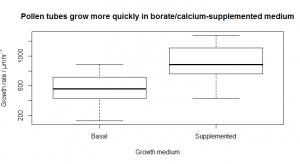pollen.tubes<-read.csv( "H:/R/pollen_tubes.csv" )
var.test( Growth.Rate ~ Medium, data = pollen.tubes )
        F test to compare two variances
data:  Growth.Rate by Medium
F = 0.7309, num df = 14, denom df = 14, p-value = 0.5653
alternative hypothesis: true ratio of variances is not equal to 1
95 percent confidence interval:
0.2453878 2.1770768
sample estimates: ratio of variances
0.7309091
t.test( Growth.Rate ~ Medium, data = pollen.tubes, var.equal=TRUE )
       Two Sample t-test data:
Growth.Rate by Medium
t = -3.7345, df = 28, p-value = 0.0008522
alternative hypothesis: true difference in means is not equal to 0
95 percent confidence interval:
-514.1032 -149.8968
sample estimates:
mean in group Basal       mean in group Supplemented
559.3333                  891.3333
tapply( pollen.tubes$Growth.Rate, pollen.tubes$Medium, mean )
       Basal        Supplemented
559.3333     891.3333
tapply( pollen.tubes$Growth.Rate, pollen.tubes$Medium, sd )
       Basal        Supplemented
223.7389     261.7037
# Plot the data with suitable title
plot(
Growth.Rate ~ Medium,
data = pollen.tubes,
xlab = "Growth medium",
ylab = expression("Growth rate / µm " * hr^-1),
main= "Pollen tubes grow more quickly in borate/calcium-supplemented medium"
)
1. The Piprecision pipettes have a variance at least twice as high as that of the Accurette model (F=0.23, p=0.00016), indicating the Acurrette pipettes are more precise. No difference in accuracy was observed (t=0.48, p=0.063) across the two brands.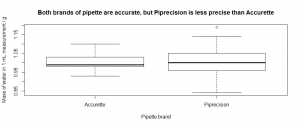pipette.check<-read.csv( "H:/R/pipette_check.csv" )

var.test( Mass ~ Brand, data=pipette.check )
        F test to compare two variances
data:  Mass by Brand
F = 0.2298, num df = 29, denom df = 29, p-value = 0.0001639
alternative hypothesis: true ratio of variances is not equal to 1
95 percent confidence interval:
0.1093666  0.4827644
sample estimates:
ratio of variances
0.2297789
t.test( Mass ~ Brand, data=pipette.check )
        Welch Two Sample t-test
data:  Mass by Brand
t = -0.4835, df = 41.659,
p-value = 0.6313
alternative hypothesis: true difference in means is not equal to 0
95 percent confidence interval:
-0.03967369  0.02434036
sample estimates:
mean in group Accurette  mean in group Piprecision
0.999000                   1.006667
tapply( pipette.check$Mass, pipette.check$Brand, mean )
  Accurette  Piprecision
0.999000     1.006667
tapply( pipette.check$Mass, pipette.check$Brand, sd )
  Accurette  Piprecision
0.03754078  0.07831560
# Plot the data with suitable title
plot(
Mass ~ Brand,
data = pipette.check,
xlab = "Pipette brand",
ylab = "Mass of water in 1 mL measurement / g",
main="Both brands of pipette are accurate, but Piprecision is less precise than Accurette"
)
1. power.t.test takes exactly four of a sample size per group (n), a difference in means (delta), a power (power = 1 − β), a standard deviation (sd), and a significance level (sig.level = α) and returns the fifth parameter. We want the difference in means here, which turns out to be 0.0119, which is a very substantial fraction of the growth rate in the control (0.02), i.e. we would only be able to reliably detect a difference in growth rate if the silver causes the growth rate constant to (slightly more than) halve in value. This is why doing power calculations is worthwhile!
power.t.test( n=4, power=0.8, sd=0.005, sig.level=0.05 )
Two-sample t test power calculation
n = 4
delta = 0.01190707
sd = 0.005
sig.level = 0.05
power = 0.8
alternative = two.sided
NOTE: n is number in *each* group

Next up… Linear regression.

## Comparison of variances: the F test

An F test is used to compare the variances of two data sets:

• As it is used to compare variances, the dependent data must – by definition – be numeric.
• As it is used to compare two distinct sets of data, these sets represent the two levels of a factor.

The test statistic we use to compare the variances of the two data sets is called F, and it is defined very simply: F is the larger of the two variances divided by the smaller.$F = \frac{s_1}{s_2}$
The reference distribution for the F test is Fisher’s F distribution. This reference distribution has two parameters: the number of degrees of freedom in the first data set, and the number of degrees of freedom in the second data set.

An F test compares the F statistic from your experimental data with the Fisher’s F value under the null hypothesis for the given degrees of freedom. The p value of the test is the probability of obtaining a test F statistic at least as extreme as the one that was actually observed, assuming that the null hypothesis (“the variances are equal”) is true. i.e. the p value is the probability of observing your data (or something even more extreme), if the variances really are equal.

The comparison is only valid if the sample data sets are:

• Both representative of the larger population. The larger the data set, the more likely this is to be true; however, it is also critical that the data be collected in an unbiased way, i.e. with suitable randomisation.
• Both normally distributed. If you plot the data as box-and-whisker plots, gross deviations from normality are often obvious. You can also use normal quantile-quantile (QQ) plots to examine the data: help(qqnorm)
• Independent of one another, i.e. there is no reason to suppose that the data in the first set are correlated with the data in the second set. If the data represent samples from the same organism at two different times, there is every reason to suppose they will be correlated. If the data represent paired samples, e.g. from one male child and from one female child, repeated across many families, there is again every reason to suppose they will be correlated.

Do not use an F test if these assumptions are broken.

In R, an F-test is performed using var.test(). Here we generate thirty items of random normal data using rnorm() and compare two such data sets. The output of your code will differ as the data is random:

dataset1<-rnorm(30)
dataset2<-rnorm(30)
var.test( dataset1, dataset2 )
        F test to compare two variances
data: dataset1 and dataset2
F = 1.7706, num df = 29, denom df = 29, p-value = 0.1298
alternative hypothesis: true ratio of variances is not equal to 1
95 percent confidence interval:
0.8427475  3.7200422
sample estimates:
ratio of variances
1.770609

Here the p value is greater than 0.05, so we say the difference in variances is not significant.

Like plot(), it is usually more convenient to use the ‘modelled-by’ tilde ~ operator to perform F tests within named data frames, rather than supplying the test with two vectors of data. Here we use the dog_whelks.csv data again.

dog.whelks<-read.csv( "H:/R/dog_whelks.csv" )
var.test( Height ~ Exposure, data = dog.whelks )
        F test to compare two variances
data:  Height by Exposure
F = 0.9724, num df = 25, denom df = 29, p-value = 0.9504
alternative hypothesis: true ratio of variances is not equal to 1
95 percent confidence interval:
0.4540033 2.1297389
sample estimates:
ratio of variances
0.9724247

“Do an F test on the data in the dog.whelks data frame, using Height as the dependent variable, and grouping the data into two sets according to the Exposure factor”

Exercises

See the next post on the t test.

## Organism of the week #19 – Bird-brained humans

Most people who want to attract birds to their gardens put out bird-seed, or – if they’re really adventurous – mealworms. However, on the East London dockside where I lived until recently, one of the residents strewed the quayside with livers in December last year. Was this was a deliberate ploy to attract carrion crows for bird-on-variety-meat action? I don’t know, but whether or not this was the intention, it was certainly the result.

Crows have a reputation as being very intelligent. I wouldn’t argue against this, but it’s interesting that intelligence is something humans tend to see in any animal that behaves somewhat similarly to them. As humans are highly social apes, most of the important things in their environment are other humans; and much of their brain-work is therefore directed at predicting (and outwitting) other humans.

Above all else, humans consider their mental talents the sine qua non of human-ness: any old sparrow can walk on two legs, and even a bloody rat can carry off the last-chicken-in-the-shop look, but solving crossword puzzles and finding new ways of fucking over your conspecifics – now that’s what being human really means.

So perhaps it’s not terribly surprising that the animals humans see as intelligent – dolphins, chimps, dogs, crows, ‘even’ bees – are those that live in complex social groups, where knowing how to interpret and predict the bahaviour of others is an important survival skill. Their communication, prediction, and manipulation remind humans of their own dubious talents. Humans delight in the familiarity they see in the activities of other intelligent animals, whilst smugly assuming that their human world is an tapestry by which the scanty threads of bird-brains pall.

But it’s all survival, adaptation, drift. Species eke out a living in the niche in which their find themselves. The human niche is different from that of a liver-pecking crow, and the talents they require are different. But it takes a human to reinterpret ‘different’ as ‘better’. The real sine qua non of human-ness is self-aggrandisement, and compared to soaring on wings of midnight, that’s not a terribly edifying talent.

## Descriptive statistics

Statistics can be used to describe a data set, or they can be used to infer how well a model fits a data set. In this post, we’ll look the the former kind of statistics, and how to extract them from R. The remaining posts in this series will mostly deal with statistical tests and modelling.

Descriptive statistics should usually give a measure of the central tendency of a data set (the mean and/or median), and of the variability of the data set (the range, quartiles, variance and/or standard deviation).

Consider the following data set:

# Number of students in lectures on successive days
students <-c(93, 95, 86, 76, 58, 77, 44, 107, 96, 95, 67, 67, 58, 87, 94, 100, 86)

Descriptive statistics should usually be accompanied by a graph that shows the data clearly to the reader in the most appropriate format for eye-balling. This set of data can be shown as a histogram in R using:

hist( students )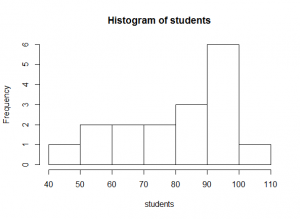The commonest ways of summarising a sample’s central tendency are to calculate the mean or the median of the sample.

A sample’s arithmetic mean (x̅) is the sum of all the values in the sample divided by the number, n, of items in the sample:$\bar{x} = \frac{\sum_{i=1}^{n} x_i }{ n }$
mean( students )
 81.52941

Although the arithmetic mean is frequently used to show the central tendency of a data set, you must take care in its interpretation. The mean number of students in lectures was 81, but you can see in the histogram above that on most days, the number of students fell between 90 and 100. This is because there is a large tail of low values in the distribution: the distribution is negatively skewed.

# Arithmetic mean, the hard way, using length() and sum()
total<-sum( students )
n<-length( students )
total/n
 81.52941

The usual measure of spread that goes with a mean, is the sample’s variance (s2). The variance is the sum of squares (SS) of the differences between each item in the sample and the mean, divided by the degrees of freedom remaining in the data set, i.e. you take each item in the data set, subtract the mean from it; square the result, add all of those squared differences up, and divide the total by the number of degrees of freedom.$s^2 = \frac{ \sum_{i=1}^{n} (x_i - \bar{x} )^2 }{ n-1 }$

Before you ask, the number of degrees of freedom in a data set is its size, n, minus the number of parameters (means, slopes, intercepts, etc.) you have estimated from it. If you estimate the mean of a set of data of size six, you remove one degree of freedom from it, and have only five left. The first five data points can take any value you like, but the value of the sixth point is constrained because the mean of all six points is known, and therefore the value of the sixth data point is also known.

var( students )
 306.7647

The standard deviation, s, is the square root of the variance. It has the advantage of having the same units as the mean, so is a useful measure of how far a ‘typical’ data point in your sample may lie from the mean of the sample.

sd( students )
 17.5147

Another measure of central tendency is often more useful when distributions are noticeably asymmetric: a sample’s median is the middle value in the ordered set of data (or the mean of the two middle samples if n is even).

median( students )
 86

The median is shown very clearly on a box-and-whisker-plot:

boxplot( students )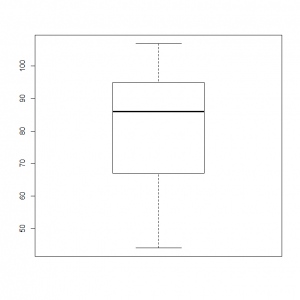In a box-and-whisker plot:

• The black bar represents the median.
• The box represents the interquartile range (25% to 75% of the data).
• The whiskers represent the full data range excluding any data more than 1.5 times larger than the upper quartile value, or 1.5 times smaller than the lower quartile.
• The dots represent any outlying data (i.e. those data excluded by the 1.5 criterion above). There are no outliers in this particular data set, but we’ll see them in later boxplots.

The range is the difference between the largest and smallest values, and the quantiles are the values at some fraction through the ordered data set, often at [0, ¼, ½ , ¾, 1] (quartiles) or at 1% intervals (percentiles).

range( students )
  44 107
quantile( students )
    0%  25% 50%  75% 100%
44   67  86   95  107
summary( students )
   Min. 1st Qu.  Median    Mean 3rd Qu.    Max.
44.00   67.00   86.00   81.53   95.00  107.00

# Exercises

Give summary statistics for the following data set

1. The file daisy_capitulae.csv contains data on the number of daisy capitulae (‘flowers’) in a larger number of quadrat samples in a field. Give some descriptive stats and a handy histogram to summarise it. Write this as prose: never just paste the raw text output of R into a document.
2. Create 10 000 normal data points using rnorm(), with mean 5 and standard deviation 2, and plot a histogram of them. help(rnorm) for the syntax.

1. In each square metre quadrat, the number of capitulae ranged from 2 to 24, with a median of 12.5. The mean number of capitulae was also 12.5(±5.6 s.d.). The histogram below show little evidence of skew, and the number of capitulae per square metre appears to be normally distributed.
daisy.capitulae<-read.csv( "H:/R/daisy_capitulae.csv" )
summary( daisy.capitulae )
Capitulae
Min.   : 2.00
1st Qu.: 9.00
Median :12.50
Mean   :12.47
3rd Qu.:15.25
Max.   :24.00
names(daisy.capitulae)
 "Capitulae"
sd( daisy.capitulae$Capitulae )  5.556182 hist( daisy.capitulae$Capitulae,
breaks = 5,
xlab   = "Capitulae",
main   = "Daisy capitulae per square metre quadrat"
)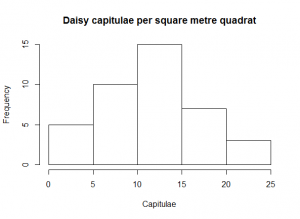1. Ten thousand normal data points
hist( rnorm(10000, 5, 2) )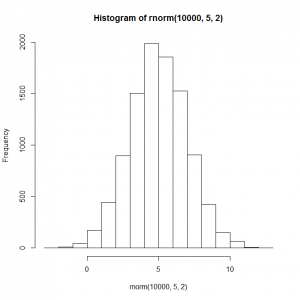Next up… Statistical testing.

## Organism of the week #18 – Feeling rotten

It’s been ages since I did one of these, and today’s will not be terribly long or exciting, for the reason that is the very title of this post. Whilst bumbling around in the fridge looking for something bland and bowel-friendly, I chanced upon a lemon that had fallen into a oubliette in the bottom of the salad drawer. Perhaps it’s just the sickness talking, but I found it rather beautiful:

I currently feel much as this lemon does – thoroughly rotten. Hopefully whatever it is that ails me will not result in my needing a prescription for the famous product of a close relative of the mould growing on this lemon.

## Plotting data

You can plot data using plot(). This can be used in several ways. The simplest is to plot some numeric x-values against some numeric y-values using:

plot( x.variable.vector, y.variable.vector )

For example:

x<-c( 1:10 )
y<-1 + 2*x
plot( x, y )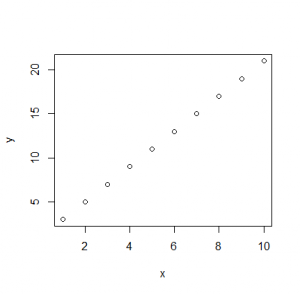However, for data you have imported into a data frame, the x and y variables are now named columns in a data frame, rather than separate vectors. To plot data in data frames, there are two options. The first is to use the dollar $syntax to access the vector of data within the data frame (here sycamore_seeds.csv) by its column name: sycamore.seeds<-read.csv( "H:/R/sycamore_seeds.csv") # You'll need to use whatever column names you chose: names( sycamore.seeds )  "wing.length" "descent.speed" plot( sycamore.seeds$wing.length, sycamore.seeds$descent.speed )The second is to use: plot( y.name ~ x.name, data = your.data.frame ) You can read the tilde ~ as modelled on. We’ll come across many other uses of the modelled-on tilde in later posts. plot( descent.speed ~ wing.length, data = sycamore.seeds ) This can be read as: “Using the data in the sycamore.seeds data frame, use the descent.speed column as the dependent variable, and model it using the wing.length column as the independent variable” As both variables are numeric, R will assume you want to plot() this model as a simple x,y-scatterplot: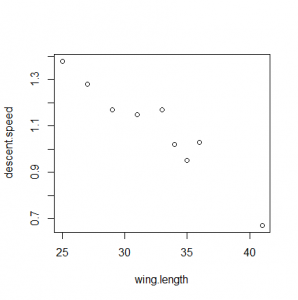Graphs ought to have better axis labels and more descriptive titles than this, which you can also control using name=value named argument pairs, namely, xlab, ylab and main: plot( descent.speed ~ wing.length, data = sycamore.seeds, xlab = "Wing length / mm", ylab = expression("Descent speed" / m*s^-1), main = "Sycamore seeds with longer wings fall more slowly" ) These labels can either be "simple strings" or they can be an expression(). Where possible, it’s easiest to use strings. However, you won’t have any choice but to use expression() if you need super/sub scripts or italic text. An expression() can include any number of the following components (demo(plotmath) for more): • "A string" • something^superscripted • something[subscripted] • x-an*algebraic/expression+y • "A string " * " masquerading as a multiplication using a * to juxtapose two items" • "A string containing a micro µ sign: you can use ASCII characters freely" • greek*symbols/pi*get-transcribed*alpha • italic("Latin name for example")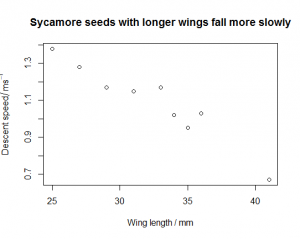Most graphs also support options such as: • Differently coloured data points: col="red" • Different plot characters: pch=15 (see help(points) for details) These and many other graphical options are explained in help(par). For data where the dependent variable is numeric, and the independent variable is categorical, R will plot the data as a box-and-whisker plot rather than an x,y-scatterplot. You can also force R to do this using boxplot() with the same parameters. dog.whelks<-read.csv("H:/R/dog_whelks.csv") boxplot( Height ~ Exposure, data = dog.whelks, xlab = "Beach", ylab = "Shell height / mm", main = "Dog whelks have taller shells on sheltered beaches" )In a box-and-whisker plot: • The black bar represents the median • The box represents the interquartile range (25% to 75% of the data) • The whiskers represent the full data range excluding any data more than 1.5 times larger than the upper quartile value, or 1.5 times smaller than the lower quartile. • The dots represent any outlying data (i.e. those data excluded by the 1.5 criterion above). You might want to base your graphing code on the template below, with simple strings for all the labels: DATAFRAME.NAME<-read.csv("H:/R/NAME_OF_FILE.csv") plot( YYY ~ XXX, data = DATAFRAME.NAME, xlab = "X-AXIS LABEL / UNITS", ylab = "Y-AXIS LABEL / UNITS", main = "GRAPH TITLE" ) You can then prettify the labels as necessary with expression()code to replace e.g. ylab = "k / per second" with ylab = expression("k"/s^-1) …as your confidence improves. Start simply, and then fettle the code until you get exactly the effect you want. If your main title starts to become too long, you can insert a line-break with a “\n” character. (“n” for “newline”) main = "GRAPH TITLE THAT GOES ON AND ON AND ON\nAND ON AND ON AND ON" You’ll probably want to put some of your graphs into other documents at some point. The simplest way to do this is to right-click the graph, copy it as a bitmap, and then paste that into the destination (or into an image editor and then save as a PNG or similar). However, this has two limitations. Firstly, the file will be a raster image of low resolution (72 dpi) which is good enough for web imagery, but not good enough for publication or large conference posters. Secondly, the axis labels and main title are at a relatively small font-size; again, fine for the web, but difficult to read on a projected PowerPoint slide or similar. Solutions to these problems. The first can be avoided by saving the plot directly from R as a scalable vector graphic (SVG) using svg(), which can be enlarged (or shrunk) to any degree without losing resolution. These can be pasted directly into Word and PowerPoint documents from Office 2016 onwards. The second can be worked around by scaling up the font-size with the various cex options (use help(par) to find out about these), unfortunately, one side-effect of this is that the axis labels can end up sliced-off the edge of the image because the margins around the plot are too narrow: this itself can be worked around by modifying the mar options. This all seems rather complicated for something as simple as generating a publication-ready image, and it is. Ho hum. Here’s a template you can modify to generate SVGs of a reasonable size (A4), with reasonable font-size, and with a left margin that doesn’t slice off the y-axis label. cricket.chirps<-read.csv( "H:/R/cricket_chirps.csv" ) # svg() sets up a printer device - there's also a pdf() and other handlers you might use too # Any calls to plot() from here on will be sent to the device rather than to the screen # The width and height are in inches; here I put them in mm with the relevant conversion factor svg( filename="H:/cricket_chirps.svg", width=298/25.4, height=210/25.4 ) # Get the default margins for plots, add a larger buffer to the left, and set the margins # to these new values. You could combine these two lines easily if you want mar.default <- par( 'mar' ) par( mar = mar.default + c( 0, 2, 0, 0 ) ) # The call to plot() now send to the SVG rather than to the screen # The cex options increase the relative font-size from the default (1) # cex affects the plots, cex.axis the numbers/etc., on the axes, # cex.lab affects the axis titles (i.e. "Temperature / °C"), and cex.main # would have changed the overall title size, were this graph to have had one plot( Frequency ~ Temperature, data = cricket.chirps, xlab = "Temperature / °C", ylab = "Frequency / Hz", cex = 2, cex.axis = 2, cex.lab = 2 ) # When we're done, we must call dev.off() or we'll continue to send plots to the file # rather than the screen dev.off() # Exercises Plot the following graphs, giving them suitable labelling. 1. The file tomato_pollination.xlsx gives data on fruit yield (number of fruits per plant) for two different kinds of pollination: by-hand, and simple spraying with water. Plot the data appropriately. You’ll need to modify the format of the file and save it as a CSV first. 2. The file reaction_rate.csv gives data on the absorbance of an enzyme-catalysed assay at 405 nm over time (min). Plot the data appropriately. Once you have this working with a simple “Absorbance (405 nm)” for the y-axis label, change the y-axis label to “A405” (with a subscript) using expression(). 3. The file fly_agarics.csv gives the number of fly agaric (Amanita muscaria) basidiocarps per hectare in two different kinds of woodland. Try a simple “Basidiocarps per hectare” style y-axis label, and just call them fly-agarics in the main title. Once you’ve done this, see if you can use expression() to fettle an italic Amanita muscaria into the main title, and a y-axis label that says “Basidiocarps / ha-1” with a superscripted minus-1. 4. The file enzyme_kinetics.csv gives the velocity of the enzyme acid phosphatase (µmol min−1) at different concentrations of a substrate, NPP (mM). After you’ve written the code for the simple graph, modify the code so set the minimum and maximum axis values using the ylim and xlim options. These both expect a two-element vector c( minimum.value, maximum.value ). The x-axis should go from 0 to the maximum x-value; the y-value should go from 0 to 10 (which is the vmax asymptote of this hyperbolic data set).  xlim = c( 0, 20 ) xlim = c( min(DATAFRAME.NAME$XXX), max(DATAFRAME.NAME$XXX) ) ylim = c( 0, max(DATAFRAME.NAME$YYY) )
1. The file asellus_gills.csv gives the number of gill movements per minute for water lice (Asellus sp.) in stagnant and oxygenated water. Make sure the Latin name in the main title is italicised.

1. Tomato pollination plot. The tomato_pollination.csv file will need to look something like the table below.
 Yield Pollination 33 Sprayed 28 Sprayed 56 Sprayed … … 46 Hand pollinated 42 Hand pollinated 63 Hand pollinated … …
tomato.pollination<-read.csv("H:/R/tomato_pollination.csv")
plot(
Yield ~ Pollination,
data = tomato.pollination,
xlab = "Pollination method",
ylab = "Yield / fruits per plant",
main = expression("Hand-pollination has no advantage over spraying in increasing tomato plant fruit yield")
)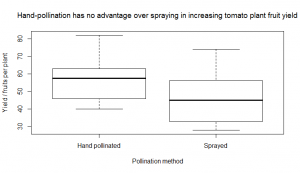1. Reaction rate plot
# Simple version, no expression() malarkey

plot(
A405 ~ t,
data = reaction.rate,
xlab = "t / min",
ylab = "Absorbance (405 nm)",
main = "Accumulation of product in the assay is linear for 30 min"
)

# More complex version with subscript

plot(
A405 ~ t,
data = reaction.rate,
xlab = "t / min",
ylab = expression(A),
main = "Accumulation of product in the assay is linear for 30 min"
)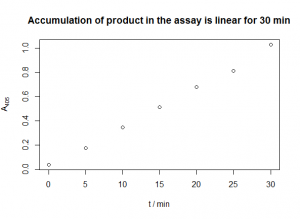1. Fly agaric plot.
# Simple version, no expression() malarkey

plot(
Basidiocarps ~ Woodland,
data = fly.agarics,
xlab = "Woodland type",
ylab = "Basidiocarps / per hectare",
main = "Fly agaric basidiocarps are more common in birch woodland"
)

# More complex version, with italics and superscripts

plot(
Basidiocarps ~ Woodland,
data = fly.agarics,
xlab = "Woodland type",
ylab = expression("Basidiocarps"/ha^-1),
main = expression(italic("Amanita muscaria") * " basidiocarps are more
common in birch woodland")
)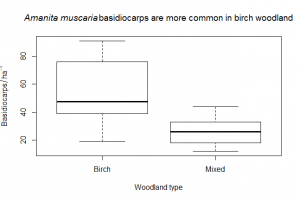1. Enzyme kinetic plot. The y-label is a bit tricky: ideally, you’d want to be able to write expression(v/µmol*min^-1) and perhaps this is what you tried. Unfortunately, the * in expression() just juxtaposes symbols, ignoring (and removing) any whitespace, even when it’s significant. Hence we have to add it manually inside a string "µmol ".
# Simple version with default limits

plot(
v ~ S,
data = enzyme.kinetics,
xlab = "[NPP] / mM",
ylab = expression(v/"µmol " * min^-1),
main = "Acid phosphatase shows saturation kinetics on the substrate NPP",
ylim = c(0,10),
xlim = c(0,max(enzyme.kinetics$S)) ) # Complex version, setting the limits nicely enzyme.kinetics<-read.csv("H:/R/enzyme_kinetics.csv") plot( v ~ S, data = enzyme.kinetics, xlab = "[NPP] / mM", ylab = expression(v/"µmol " * min^-1), main = "Acid phosphatase shows saturation kinetics on the substrate NPP", ylim = c(0,10), xlim = c(0,max(enzyme.kinetics$S))
)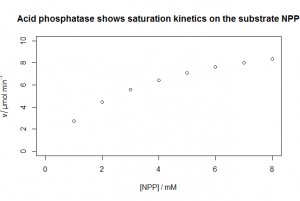1. Asellus gill movement plot.
asellus.gills<-read.csv("H:/R/asellus_gills.csv")
plot(
Gill.movements ~ Water.quality,
data = asellus.gills,
xlab = "Water quality",
ylab = expression("Gill movements "/ min^-1),
main = expression("Water fleas (" * italic("Asellus") * "sp.) breathe harder in stagnant water")
)

## Formatting data

Although you will occasionally type data directly into R, more often than not, you will have a spread-sheet containing data which you want to import. R can easily import data from a comma-separated variable (CSV) file. If you open a CSV file using a text-editor, you will see that they look something like this inside (this is dose_response.csv):

C,mu
0.125,0.69
0.25,0.68
0.5,0.687
1,0.63
2,0.467
4,0.252
8,0.091
16,0.021

The first row contains labels for the columns, and the remaining rows contain the data. The pair of labels, and the pairs of data, are each separated by a comma.

R can import CSV files directly using the read.csv() function. Functions in R always have their arguments (i.e. the numbers, vectors, etc., they act upon) enclosed by a pair of ( parentheses ). Here we assign the contents of the file to a variable called dose.response.data:

dose.response.data<-read.csv( "H:/dose_response.csv")
print( dose.response.data )
       C    mu
1  0.125 0.690
2  0.250 0.680
3  0.500 0.687
4  1.000 0.630
5  2.000 0.467
6  4.000 0.252
7  8.000 0.091
8 16.000 0.021

Note the paired " quotes " around the file-path: in R, strings of characters like this must always be enclosed in quotes.

I will conventionally assume you have created a folder called R or similar in your home-directory, in which you save and store all of your R data and scripts:

dose.response <-read.csv( "H:/R/dose_response.csv")

R uses the UNIX convention of a forward-slash / (rather than the Windows convention of a backwards slash \) to navigate down through folders in the file-system.

If for some reason you forget where you have stored a CSV file, you can browse to it by passing the function file.choose() to read.csv():

dose.response <-read.csv( file.choose() )

N.B. Under Windows, the .csv part of the file-name (the file-extension) will usually be ‘helpfully’ hidden. You can (and should) unhide them for all files (in Win7 Explorer: Organize→ Folder and Search Options→View→uncheck ‘Hide extensions for known file types’).

The read.csv() function will import the data from the CSV file into an object called a data frame. This contains several named vectors (i.e. the columns). Although you will usually import them, you can create data frames manually by grouping vectors together using the data.frame() function:

conc.vector<-c( 0.125, 0.25, 0.5, 1, 2, 4, 8, 16 )
mu.vector<-c( 0.69,0.68,0.687,0.63,0.467,0.252,0.091,0.021)
manual.dose.response <-data.frame( C=conc.vector, mu=mu.vector )
print( manual.dose.response )

Note the option.name=option.value syntax: this is the standard way to pass named arguments to an R function.

To create an importable CSV file from your own data in an Excel spread-sheet, you need to:

• Lay the data out in an otherwise empty workbook. If you are using a European version of Excel, you should change the options to use a full stop as the decimal separator, not a comma (in Excel, press F1 and search for “decimal separator” to find out how to do this).
• Use the top row to give meaningful labels to the columns. Avoid spaces, commas and other punctuation characters in these labels, as these will be used as the column names in R, and these should follow the same conventions as for variables. If you need to use anything other than letters, you can use a full-stop or underscore for clarity e.g. conc.mM or mu_perhour.
• Use “Save As” to save the file as a sensibly named “CSV (Comma delimited) (*.csv) file” (I’ll assume you’re saving to and loading from H:/R ).  Don’t just call it data.csv or you’ll rapidly get confused about what “data” it contains! Again, avoid spaces and similar punctuation: you can use a full-stop or an underscore instead.
• A CSV file is a single list of plain-text values, so Excel will probably moan about incompatible things because your spread-sheet may contain multiple worksheets, formulae, bold text, etc. It’s usually fine to just click ‘OK’.
• Close Excel, and you should now be able to import the new CSV file into R directly.
• Once you’ve imported the data, check the column headings and the data have imported and appear as you expect:
names( dose.response.or.whatever.the.data.frame.is.called)
print( dose.response.or.whatever.the.data.frame.is.called)

In the table below, each row corresponds to a person whose height and mass you have measured. Data of the sort in the table below is continuous: both the height and mass values are numeric, and the values they can take are not restricted to integer values. The correct way to arrange such data in a spread-sheet for import into R is the ‘intuitive’ way, where each row corresponds to a datum.

 Height / m Mass / kg 1.60 65 1.69 70 1.54 72 1.81 85

However, the ‘intuitive’ way of arranging categorical data is generally not how R needs it arranged:

Heights of smokers and non-smokers (m)

 Smokers Non-smokers 1.60 1.61 1.69 1.90 1.54 1.42 1.81 1.55

Each row contains data from two different individuals, and there is no particular reason that the smoker who is 1.60 m tall should be paired with the non-smoker who is 1.61 m tall. There’s also no particular reason why the columns should be the same length either. This is not an appropriate way to lay out categorised data for import into R. In R, you need to tabulate the data as shown below with one row for each ‘individual’ record. The levels (here, Y or N, but they could be called Smoker and Non.Smoker or whatever you prefer) of each factor (just one here: Smokes) are listed in the same way as you would if they were continuous data.

 Height Smokes 1.60 Y 1.69 Y 1.54 Y 1.81 Y 1.61 N 1.90 N 1.42 N 1.55 N

The same applies even if the data set is entirely categorical: each row should be an ‘individual’, not a count. A contingency table of count data like this is not generally what you want to import into R:

 Male Female Genderqueer Left-handed 1 2 0 Ambidextrous 0 1 0 Right-handed 2 3 1

R can produce a contingency table from the raw data (we’ll see this later), but if you’re importing the raw data, there should be a single row for each ‘individual’, as shown below:

 Gender Handedness Height Female L … Female L Female A Female R Female R Female R Genderqueer R Male L Male R Male R

If you ever get stuck wondering how to format categorical data, imagine that for each ‘individual’ you have measured, there is an extra item of continuous data to add too (e.g. the height of the people in the table above, as shown by the italicised column heading and empty cells). Adding that extra data to the table must be simple matter of pasting it into a new column of the same length, otherwise the format of the table you have produced is wrong.

# Exercises

Perform the following data import in R.

1. Edit and save the file sycamore_seeds.xlsx into a form in R can import (call it sycamore_seeds.csv). This shows the length of a sycamore seed’s wing compared to the speed with which it descends when dropped. Read the data into R, and check it has imported correctly.
2. R contains a lot of example data sets, which you can obtain a list of using data(). R is also capable of writing CSV files just as easily as it can read them: try help(write). Output the DNAse ELISA assay data to a file called dnase_elisa.csv.
3. Edit and save the file dog_whelks.xlsx into a form in R can import (call it dog_whelks.csv). The data shows the shell-height of dog-whelks on sheltered vs. exposed shores. Read the data into R, and check it has imported correctly.

1. Your XLSX file should look something like this before you save it as sycamore_seeds.csv:
 wing.length descent.speed 25 1.38 41 0.67 27 1.28 35 0.95 36 1.03 31 1.15 34 1.02 29 1.17 33 1.17
1. Save the ELISA data:
write.csv( DNase, file="H:/R/dnase_elisa.csv" )
1. Your dog whelk XLSX file should look something like this before you save it as dog_whelks.csv:
 Height Exposure 22 Sheltered 23 Sheltered 26 Sheltered … … 15 Exposed 17 Exposed 19 Exposed … …

Next up… Plotting data.

## Kinds of data

The sort of data you want to analyse in R may come in many forms. You will often be trying to model the interaction of some independent variables (a.k.a. input, predictor or explanatory variables, the things you’d plot on an x axis) to explain the values of some dependent variable (a.k.a. output, outcome or response variable, the thing you’d put on a y axis).

Categorical (qualitative) data is data that is most clearly expressed as a one of a fixed number of levels of a factor. The factor “rock type” has three conventional levels: “igneous”, “sedimentary” and “metamorphic”. You could code these nominal levels numerically as ‘1’, ‘2’ and ‘3’, but the named levels are easier to use, and do not imply the data is ordered in the way numerical coding of the levels might. However, some kinds of categorical data are sensibly arranged in an order, like “disagree”, “neutral” and “agree”; these are ordinal data. The difference between ordinal data and true numerical data is that the interval (the ‘gap’) between the categories of pure ordinal data is impossible to quantify: is the difference between “neutral” and “agree” really the same as between “agree” and “strongly agree”, and is that question even meaningful?  Categorical data that can only take one of two values is binary data: a light-switch can be on or off; a human can be dead or alive.

Numerical (quantitative) data is data that is most sensibly expressed as a number, like human height. The key difference from ordinal categorical data is that the interval between the values of a numerical variable is consistent and meaningful: the difference between 1 apple and 2 apples is the same as the difference between 5 apples and 6 apples, but you can’t say that of the difference between having “A-levels” and “BSc” vs. “MSc” and “PhD”. Numerical data that can take a true zero value is ratio data; that which lacks a true zero is interval data. The Celcius temperature scale can take a zero value, but “temperature in Celcius” is still interval data because the zero on this scale is  arbitrary: something at 40°C is not twice as hot as something at 20°C. However, “temperature in Kelvin” is ratio data because the zero is meaningful – it indicates a complete absence of molecular motion – so something at 400 K is in a genuine sense twice as hot as something at 200 K.

Whether interval or ratio, numeric data can also be continuous (i.e. real in the mathematical sense, x ∈ ℝ) or discrete. Human height is continuous, as any positive real value is possible, but it is also bounded at the lower end by zero, as human height cannot be negative. Analysis of bounded data can be problematic if many of the data are close to one or other bound (e.g. percentage attendance in exams: likely to be 90 to 100%); in other cases, the bounds may make no practical difference to the analysis (e.g. human height: very few individuals will be close to the bounds of 0 m or 3 m). Numeric data can also be unbounded. A company’s profit is continuous and unbounded at both ends (negative profit = loss). Many continuous unbounded variables are distributed according to the normal distribution. Percentage data is a particular kind of numeric data that is strictly bounded between 0 and 100 (or between 0 and 1 if you prefer to express this as a frequency). If your dependent variable is percentage or frequency data, random variation in the variable is likely to be distributed according to the binomial distribution, and particular care must be taken in its analysis.

Numeric data can be discrete rather than continuous (i.e. an integer, x ∈ ℤ). The number of apples sold by a shop in a week is discrete and unbounded (they may “sell” a negative quantity if they buy more than they sell). Discrete numeric ratio data bounded at a true zero is count data. The number of offspring a cat has is discrete (i.e. it must be an integer), bounded count data, as a cat cannot sensibly have 2.4 kittens or minus-3 kittens. If your dependent variable is count data, random variation in the variable is likely to be distributed according to the Poisson distribution, and particular care must be taken in its analysis.

Numerical and categorical data can and do blur into one another. Both categorical and integer data are inherently discrete, and you may also take more-or-less continuous data (like grades on an exam) and deliberately transform them to ordinal categorical data by dividing them into discrete bins (A, B, C…) because it is convenient or meaningful. But you can also look at count and percentage data and see them as a large collection of binary responses: a death rate of 20% in a sample of 5 people means you’ve counted 4 dead people and 1 living person, or – equivalently – the answers “no”, “yes”, “no”, “yes”, “yes” to the question “is this one still alive?” And you can even look at continuous data as discrete data where the intervals are so small that you can’t distinguish them (or where the intervals become meaningless because quantum physics hates you).

Different kinds of statistical approach are appropriate to the analysis of different combinations of variables. For example, to test whether a continuous dependent variable (e.g. life-span) varies significantly across a categorical independent variable (e.g. handedness), then a t test might be appropriate. However, a t test would be inappropriate to test whether a continuous dependent variable (e.g. growth rate) varies significantly across a continuous independent variable (e.g. nutrient concentration): here some sort of regression would be required. It is critical that you know what sorts of test are appropriate to different kinds of data.

Certain kinds of data can be approximated as data of a different kind. It is also common to measure proxies of the data you are actually interested in: e.g. measuring optical density to quantify a number of bacterial cells; or mass to measure a number of molecules. However, you should be careful about the assumptions you are making in doing this, and you should also consider what your data analysis might be used for. The population of bacteria in a flask is really count data (1 cell, 2 cells … a trillion cells), but you might reasonably approximate this as continuous data, even though “half a cell” is not possible. Whether the data is treated as count or continuous makes no real difference in calculating how much glucose you need to add to the flask to support the bacteria: the difference in the amount needed to support 1 trillion cells versus 1 trillion and 1 is negligible. Conversely, sex in humans is continuous data, but often ends up being badly approximated as binary data. In a small sample of humans, you may not have any individuals who identify as intersex; however, they compose about 1% of the wider population, and their needs will never be noted – let alone met – if you approximate sex as binary data.

# Exercises

What kinds of data are the following?

1. Wavelength of light
2. Temperature
3. Clutch size
4. Rate of a reaction
5. Eye-colour
6. Score in Scrabble
7. Degree class
8. Ground-cover by grass in a quadrat
9. Winning side in chess
10. Whether people “love”, “like”, “meh”, “dislike” or “despise” this tutorial

1. Wavelength of light is ratio data – continuous, numeric, and bounded by a true zero. It has no upper bound, although for wavelengths you’re likely to be interested in, the boundedness will make no practical difference to the analysis.
2. Temperature is continuous numeric data. It is strictly bounded by 0 K (unless you’re doing something very odd). As we discussed above, it may be ratio or interval data depending on the units you use.
3. Clutch size (number of eggs in a nest) is count data: discrete, numeric, ratio data, bounded at 0.
4. Rate of a reaction is continuous, numeric, unbounded, ratio data: rate of reaction could be negative, i.e. products become reactants. (Quantum physicists may feel free to disagree).
5. Eye-colour is (allegedly) nominal categorical data, but this is very likely to result in a lot of arbitrary pigeon-holing.
6. Score in Scrabble is discrete numeric data, bounded at a true zero, but could probably be reasonably approximated as unbounded continuous numeric data as scores are generally quite large.
7. Degree class is ordinal categorical data (1st, 2:1, 2:2, 3rd, fail) although it is based on underlying data that may be percentages, counts, or who knows what.
8. Ground-cover by grass in a quadrat is percentage data: numeric, but strictly bounded between 0 and 100%. In theory it is continuous, but in practice, you’ll really be scoring it into discrete bins.
9. Winning side in chess is binary data: white or black (0 or 1). Unless there’s a draw…
10. A Lickert scale is ordinal categorical data.

Next up…Formatting data.

## Running R code

The R interpreter is controlled by typing in plain-text commands at its command-line prompt >|. R commands are just text, so you can prepare a chunk of them in a text-editor, and then copy-and-paste them directly into R to run them immediately. Try copying and pasting the line below at the prompt:

print("Hello world")

Lo and behold, R will spit out what you knew it would:

 "Hello world"

R comes with its own simple text-editor, which you can open using the “File…New script” menu option. You can edit R code in this box, execute everything that is currently in the editor by pressing Ctrl-A and then Ctrl-R, and save the code using “File…Save as” for later reloading and use with “File…Open script”. Avoid preparing R scripts in Word: by default, Word ‘corrects’ quote characters (“…”) to smart-quotes (“…”) which R does not understand.

Even more critically, avoid copying-and-pasting chunks of R code you do not understand: blindly shovelling data into a black-box and assuming the output is correct and meaningful will eventually lead to embarrassing catastrophe.

If you enter data into R directly by typing at the >| prompt, R will just spit it back out at you, preceded by a numeric label .

42
 42

To store a number for later use, you need to assign it to a variable, such as a, with the <-assignment operator (“gets-arrow“):

a<-42

R doesn’t output anything here, but if you want to see the value you have stored in the variable named a, you can print() it:

print( a )
 42

You can also just type:

a
 42

with the same effect. Variable names should be given meaningful names (which a probably isn’t). They must start with a letter, and should contain only letters, numb3r5, full.stops and under_scores. They are case-sensitive, so the variable nubbin is different from the variable Nubbin or the variable NUBBIN.
In R, it is very common to want to handle vectors, which are ordered lists of numbers (or of other kinds of value) stored in a variable. You can create these using the c() function (‘c’ for concatenate):

radii<-c( 1, 1.2, 3, 3.6, 7, 9 )

# The white-space between the listed items is optional, but aids readability.
# These lines starting with a hash '#' are comments. They are ignored by R
# but can be useful to explain to yourself and others what the code is doing.
# Blank lines are also ignored.

radii
 1.0 1.2 3.0 3.6 7.0 9.0

Longer vectors will be output over multiple lines, with the numerical labels indicating the index of the first item on that row. Vectors are one example of a number of different kinds of object which R can use to store different kinds of data. We’ll see data frame objects later. The usefulness of vectors becomes obvious when you find that R has functions like mean(), max() and min():

mean( radii )
 4.133333
max( radii )
 9
min( radii )
 1

You can extract an item from a vector using square brackets to index into the vector like this:

fifth.item<-radii
print( fifth.item )
 7

You can create simple vectors of integers from one number to another using the colon (:) syntax.

one.to.ten<-c(1:10)
print(one.to.ten)
   1  2  3  4  5  6  7  8  9 10

R can perform mathematical calculations on numbers and objects:

a+3
 45

If you perform a calculation on a single vector, the calculation is applied individually to each item in the vector, and the resulting values can be captured into a new vector like this:

areas<-pi*(radii^2)
areas
   3.141593   4.523893  28.274334  40.715041 153.938040 254.469005

The usual mathematical operators (+, -, *, /, ^), shorthand for scientific notation (1E6) and functions (sin(), sqrt(), exp(), log()) will do what you expect. Note that log() returns natural log, not base-10 log.
As you create vectors and other kinds of object, you will begin to fill up R’s namespace with variable names. To see what you currently have in memory, use:

ls()
  "asellus.gills"      "dog.whelks"         "enzyme.kinetics"
 "reaction.rate"      "students"           "sycamore.seeds"

To delete moribund objects use:

rm( students )

To delete everything, use:

rm( list = ls() )

It is easy to find help on R from its own HTML documentation. If you know the name of the function you want help on, you can use either of these:

help( "t.test" )
?t.test

If you don’t know the name of the function, you can search for a term in the documentation with:

help.search( "chi squared" )

The result of this search contains a link to the documentation for stats::chisq.test(), amongst other things.

When you make a mistake in R, it will tell you so:

2+lg( 3 )
Error: could not find function "lg"

It can be frustrating to find that this is due to a simple typo, particularly if this is a long line of code. However, it is easy to recall, edit and replay the last command you typed: press the ↑ arrow key to scroll through the last command(s) you typed, use the ← and → arrow keys to move to the place you want to edit, edit the text, and then press the “Enter” key to re-run the command.

# Exercises

1. The molar absorbance coefficient (ϵ) of riboflavin at 440 nm is 54×103 L mol−1 cm−1. In a cuvette with a path-length (l) of 1 cm, what is the absorbance (A) of a 10 µM solution? A = ϵ C l. Resist the temptation to do this with a calculator: use R as your calculator.
2. What is the volume (V) of an Escherichia coli cell, in cubic micrometres, if you model it as a cylinder of height (h) 2 µm and radius (r) 0.25 µm? V = π r2 h.
3. In the data below of A260 values from a protein-estimation practical, what is the largest value? The smallest value? The mean value? The median value? Create a vector called A260.sorted containing the values in ascending order so you can manually check these results. What is the thirteenth highest value? You can copy-and-paste the data below into R directly (and in so doing, you may note something helpful).
0.457, 0.314, 0.298, 0.284, 0.298, 0.42, 0.266, 0.285, 0.31, 0.288, 0.312,
0.284, 0.31, 0.255, 0.297, 0.293, 0.274, 0.253, 0.331, 0.243, 0.314, 0.269,
0.711, 0.46, 0.23, 0.314, 0.336, 0.255, 0.307, 0.243, 0.42, 0.302, 0.46,
0.297, 0.284, 0.283, 0.282, 0.231, 0.266, 0.228, 0.228, 0.402, 0.282,
0.312, 0.26, 0.247, 0.283, 0.288, 0.302, 0.252, 0.902, 0.336, 0.247,
0.231, 0.261, 0.283, 0.307, 0.457, 0.274, 0.288, 0.288, 0.461, 0.293,
0.314, 0.404
1. What do seq(0,10,2), rep(10,3) and A260[A260>0.5] do?
2. The mass of wood in a pine-tree of girth (circumference at ground-level) c, and of height h can be roughly approximated by considering the pine tree as a cone of volume V =c2 h / 12 π , and of the same density as water (i.e. 1 t m−3). What are the masses of the following trees? We haven’t covered the syntax for this explicitly, but see if you can work it out. R is a lot more intuitive than you might think.
Girth / m Height / m
1 10
3.5 18
1.8 25
9 50
1. What is the sum of all the numbers from 1 to 100?

1. Absorbance of riboflavin solution:
54E3*10E-6
 0.54
1. Volume of an Escherichia coli cell:
pi*0.25^2*2
 0.3926991
1. A260 values:
A260<-c(
0.457, 0.314, 0.298, 0.284, 0.298, 0.42, 0.266, 0.285, 0.31, 0.288, 0.312,
0.284, 0.31, 0.255, 0.297, 0.293, 0.274, 0.253, 0.331, 0.243, 0.314, 0.269,
0.711, 0.46, 0.23, 0.314, 0.336, 0.255, 0.307, 0.243, 0.42, 0.302, 0.46,
0.297, 0.284, 0.283, 0.282, 0.231, 0.266, 0.228, 0.228, 0.402, 0.282,
0.312, 0.26, 0.247, 0.283, 0.288, 0.302, 0.252, 0.902, 0.336, 0.247,
0.231, 0.261, 0.283, 0.307, 0.457, 0.274, 0.288, 0.288, 0.461, 0.293,
0.314, 0.404
)
# You'll notice you can paste this line-by-line: as R knows the expression
# isn't finished until you close the ')' parenthesis at the end, it will
# prompt you with a '+' rather than a '>' until you have entered the ')'
# These lines with hash '#' signs are also valid R syntax: R ignores
# lines starting with '#', and treats them as comments

max(A260)
 0.902
min(A260)
 0.228
mean(A260)
 0.3194769
median(A260)
 0.288
A260.sorted<-sort(A260)
A260.sorted
 0.255
print(A260.sorted)
 0.228 0.228 0.230 0.231 0.231 0.243 0.243 0.247 0.247 0.252 0.253 0.255
 0.255 0.260 0.261 0.266 0.266 0.269 0.274 0.274 0.282 0.282 0.283 0.283
 0.283 0.284 0.284 0.284 0.285 0.288 0.288 0.288 0.288 0.293 0.293 0.297
 0.297 0.298 0.298 0.302 0.302 0.307 0.307 0.310 0.310 0.312 0.312 0.314
 0.314 0.314 0.314 0.331 0.336 0.336 0.402 0.404 0.420 0.420 0.457 0.457
 0.460 0.460 0.461 0.711 0.902
1. Useful vector functions
# Construct a vector containing integers from 0 to 10, but in steps of 2
seq(0,10,2)
  0  2  4  6  8 10
# Construct a vector containing three repeats of the number 10
rep(10,3)
 10 10 10
# Return just the numbers in a vector greater than some value
A260[A260>0.5]
 0.711 0.902
1. Mass of wood in pine-trees:
c<-c(1, 3.5, 1.8, 9)
h<-c(10, 18, 25, 50)
M<-1*c^2*h/(12*pi)
# Multiplication of two vectors of the same length in R results in
# a new vector of the same length containing the item-wise products.
# R can do 'vector multiplication' in the mathematical sense too (dot/cross)
# but using a different syntax
print(M)
   0.2652582   5.8489442   2.1485917 107.4295866
1. Sum from 1 to 100
sum(c(1:100))
 5050

Next up…Kinds of data.

## Teardrops of the swan

Last year, the most marvellous thing we saw in the pond-water microscopy practical was a ciliate, and this year the prize goes to that same clade. Ciliates don’t disappoint.

This is Lacrymaria olor, the “teardrop of the swan”. It’s a predator, like the Vorticella from last year, but rather than sitting rooted to the spot, Lacrymaria is an actively swimming hunter, with an enormously extendable proboscis that it uses to capture prey: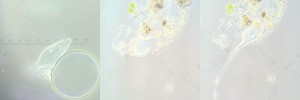Lacrymaria olor (left: worrying an air-bubble; middle: burrowed into some debris with proboscis retracted; right: ATTACK!)

Lacrymaria is only about 100 µm long in its unextended state, so although it’s just about visible to the naked eye, it’s still very, very small. Consider that the systems controlling its proboscis, its burrowing, and its hunting behaviour, are all fitted into a single cell, without benefit of a brain, or even a nervous system. Ciliates are truly wondrous beasts.